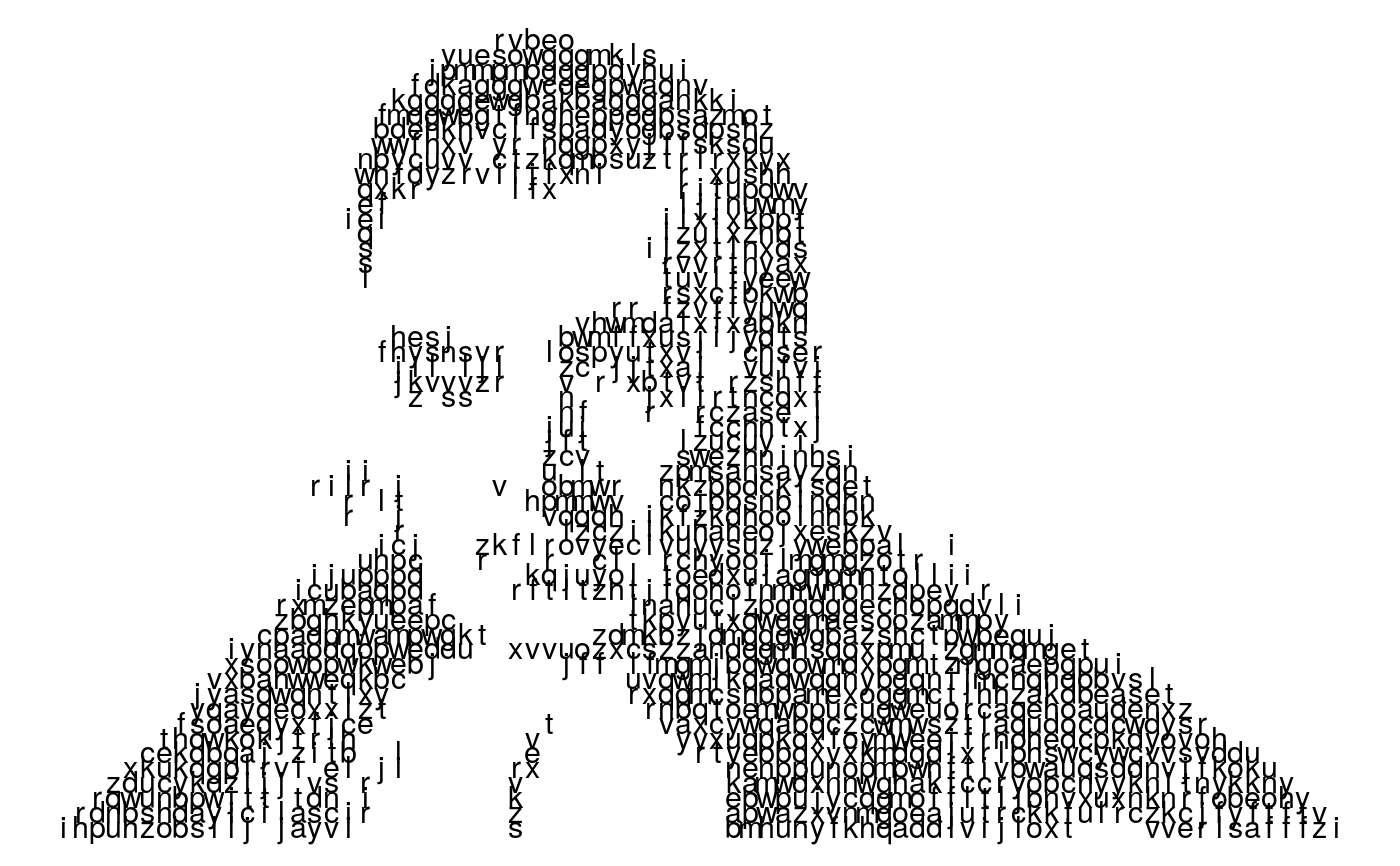Plots an ASCII character map

ascii_plot(image_map, charsize = 4)

## Arguments

image_map A tibble specifying a character map Size of the characters

A ggplot object.

## Details

A simple plotting function for a character map. It takes a tibble as input, in the form output by the ascii_map function, and plots it using ggplot2. The charsize argument allows you to customise the size of the characters in the plot

## Examples

bayes_img <- ascii_data("bayes.png")
bayes_map <- ascii_map(file = bayes_img)#> Warning: Assuming third dimension corresponds to colour#> Warning: Using a rescale value of 0.175ascii_plot(bayes_map)